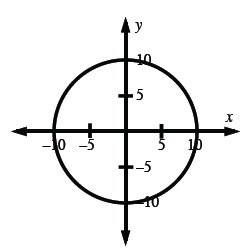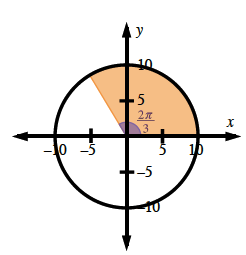### Home > CCA2 > Chapter Ch7 > Lesson 7.1.5 > Problem7-84

7-84.

Sketch a graph of $x^2 + y^2 = 100$.1. Is it a function?

Does each $x-$value on the graph of the function have only one corresponding $y-$value?

2. What are its domain and range?

Domain: $−10\le x\le10$
Range: $−10\le y\le10$

3. Draw a central angle that measures $\frac { 2 \pi } { 3 }$ radians. If you remove this wedge of the circle, how much area remains?

See the unit circle graph below.
What portion of the circle is shaded?Since $\frac{2\pi}{3}$ is $\frac{1}{3}$ of the total angle in a circle, the remaining area will be $\frac{2}{3}$ of the total area.